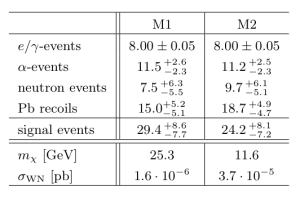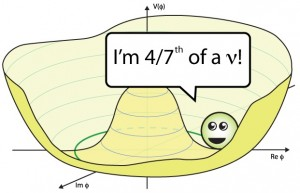## New Results from the CRESST-II Dark Matter Experiment

• Title: Results on low mass WIMPs using an upgraded CRESST-II detector
• Author: G. Angloher, A. Bento, C. Bucci, L. Canonica, A. Erb, F. v. Feilitzsch, N. Ferreiro Iachellini, P. Gorla, A. Gütlein, D. Hauff, P. Huff, J. Jochum, M. Kiefer, C. Kister, H. Kluck, H. Kraus,  J.-C. Lanfranchi, J. Loebell, A. Münster, F. Petricca, W. Potzel, F. Pröbst, F. Reindl, S. Roth, K. Rottler, C. Sailer, K. Schäffner, J. Schieck, J. Schmaler, S. Scholl, S. Schönert, W. Seidel, M. v. Sivers, L. Stodolsky, C. Strandhagen, R. Strauss, A. Tanzke, M. Uffinger, A. Ulrich, I. Usherov, M. Wüstrich, S. Wawoczny, M. Willers, and A. Zöller
• Published: arXiv:1407.3146 [astro-ph.CO]

CRESST-II (Cryogenic Rare Event Search with Superconducting Thermometers) is a dark matter search experiment located at the Laboratori Nazionali del Gran Sasson in Italy. It is primarily involved with the search for WIMPs, or Weakly Interacting Massive Particles, which play a key role in both particle and astrophysics as a potential candidate for dark matter. If you are not yet intrigued enough about dark matter, see the list of references at the bottom of this post for more information. As dark matter candidates, WIMPs only interact via gravitational and weak forces, making them extremely difficult to detect.

CRESST-II attempts to detect WIMPs via elastic scattering off nuclei in scintillating CaWO4 crystals. This is a process known as direct detection, where scientists search for evidence of the WIMP itself; indirect detection requires searching for WIMP decay products. There are many challenges to direct detection, including the relatively low amount of recoil energy present in such scattering. An additional issue is the extremely high background, which is dominated by beta and gamma radiation of the nuclei. Overall, the experiment expects to obtain a few tens of events per kilogram-year.Figure 1: Expected number of events for background and signal in 2011 CRESST-II run; from 1109.0702v1.

In 2011, CREST-II reported a small excess of events outside of the predicted background levels. The statistical analysis makes use of a maximum likelihood function, which parameterizes each primary background to compute a total number of expected events. The results of this likelihood fit can be seen in Figure 1, where M1 and M2 are different mass hypotheses. From these values, CRESST-II reports a statistical significance of 4.7σ for M1, and 4.2σ for M2. Since a discovery is generally accepted to have a significance of 5σ, these numbers presented a pretty big cause for excitement.

In July of 2014, CRESST-II released a follow up paper: after some detector upgrades and further background reduction, these tantalizingly high significances have been revised, ruling out both mass hypotheses. The event excess was likely due to unidentified  e/γ background, which was reduced by a factor of 2 -10 via improved CaWO4 crystals used in this run. The elimination of these high signal significances is in agreement with other dark matter searches, which have also ruled out WIMP masses on the order of 20 GeV.

Figure 2 shows the most recent exclusion curve for the WIMP mass, which gives the cross section for production as a function of possible mass. The contour reported in the 2011 paper is shown in light blue. The 90% confidence limit from the 2014 paper is given in solid red, alongside the expected sensivity from the background model in light red. All other curves are due to data from other experiments; see the paper cited for more information.

Though this particular excess was ultimately not confirmed, these results overall present an optimistic picture for the dark matter search. Comparison between the limits from 2011 to 2014 show an much greater sensitivity for WIMP masses below 3 GeV, which were previously un-probed by other experiments. Additional detector improvements may result in even more stringent limit setting, shaping the dark matter search for future experiments.

## Fractional particles in the sky

Title: Goldstone Bosons as Fractional Cosmic Neutrinos

Author: Steven Weinberg (University of Texas, Austin)
Published: Phys.Rev.Lett. 110 (2013) 241301 [arXiv:1305.1971]

The Standard Model includes three types of neutrinos—the nearly-massless, charge-less partners of the leptons. Recent measurements from the Planck satellite, however, find that the ‘effective number of neutrinos’ in the early universe is$N_\text{eff} = 3.36 \pm 0.34$. This is consistent with the Standard Model, but one may wonder what it means if this number really were fractional amount larger than three.Physically,$N_\text{eff}$ is actually a count of the number of light particles during recombination: the time in the early universe where the temperature had cooled enough for protons and electrons to form hydrogen. A snapshot era is imprinted on the cosmic microwave background (CMB). Particles whose masses are much less than the temperature—like neutrinos—are effectively ‘radiation’ during this era and affect the features of the CMB; see the appendix below for a rough sketch. In this way, cosmological observations can tell us about the spectrum of light particles.

The number$N_\text{eff}$ is defined as part of the ratio between photons and non-photon contributions to the ‘radiation’ density of the universe. It is normalized to count the number of light fermion–anti-fermion pairs. In this paper, Steven Weinberg points out that a light bosonic particle can give a fractional contribution to this counting. First of all, fermionic and bosonic contributions to the energy density differ by 7/8ths due to the difference between Fermi and Bose statistics. Secondly, a boson that is its own antiparticle picks up an additional 1/2, so that it looks like a light boson should contribute$\displaystyle \Delta N_\text{eff} = \frac{1}{2} \left(\frac{7}{8}\right)^{-1} = \frac{4}{7} = 0.57.$

We have two immediate problems:

1. This is still larger than the observed mean that we’d like to hit,$\Delta N_\text{eff} = 0.36$.
2. We’re implicitly assuming a new light scalar particle but quantum corrections generically make scalars very massive. (This is the essence of the Hierarchy problem associated with the Higgs mass.)

To address the second point, Weinberg assumes the new particle is a Goldstone boson—scalar particles which are naturally light because they’re associated with spontaneous symmetry breaking. For example, the lowest energy state of a ferromagnet breaks rotational symmetry since all the spins align in one direction. “Spin wave” excitations cost little energy and behave like light particles. Similarly, the strong force breaks chiral symmetry—which relates the behavior of left- and right-handed fermions. The pions are Goldstone bosons from this breaking and indeed have masses much smaller than other nuclear states like the proton. In this paper, Weinberg imagines that a new symmetry is broken spontaneously and the resulting Goldstone boson is the light state which can contribute to the number of light degrees of freedom in the early universe,$N_\text{eff}$.

This set up also gives a way to address the first problem, how do we reduce the contribution of this particle,$\Delta N_\text{eff}$, to better match what we observe in the CMB? One crucial assumption in our estimate for$\Delta N_\text{eff}$ was that the new light particle was in thermal equilibrium with neutrinos. As the universe cooled, the other Standard Model particles became too heavy to be produced thermally and their entropy had to go towards heating up the lighter particles. If the Goldstone boson fell out of thermal equilibrium too early—say, its interaction rate became too small to overcome the expanding distance between it and other particles—it won’t be heated by the heavy Standard Model particles. Because only the neutrinos are heated, the Goldstone contributes much less than 4/7 to$N_\text{eff}$. (A sketch of the argument is in the appendix below.)

Weinberg points out that there’s an intermediate possibility: if the Goldstone boson just happens to go out of thermal equilibrium when only the muons, electrons, and neutrinos are still thermally accessible, then the only temperature increase for the neutrinos that isn’t picked up by the Goldstone comes from the muon. The expression for the entropy goes like$\displaystyle s \sim T^3 \left(\text{Photon}\right) + \frac{7}{8} T^3 \left(\text{SM}\right)$

where “SM” refers to the number of Standard Model particles: a left-handed electron, a right-handed electron, a left-handed muon, a right-handed muon, and three left-handed neutrinos. (See this discussion on handedness.) The famous 7/8 shows up for the fermions. In order to conserve entropy when we lose the two muons, the other particles have to heat up by a factor of $latex (57/43)^{1/3}$. Meanwhile, the Goldstone boson temperature stays constant since it doesn’t interact enough with the other particles to heat up. The contribution of the Goldstone to the effective number of light particles in the early universe is thus scaled down:$\displaystyle \Delta N_\text{eff} = \frac{4}{7} \times \left(\frac{43}{57}\right)^{4/3} = 0.39,$

This is now quite close to the$\Delta N_\text{eff} = 0.36 \pm 0.34$ measured from the CMB. Weinberg goes on to construct an explicit example of how the Goldstone might interact with the Higgs to produce the correct interaction rates. As an example of further model building, he then notes that one may further construct models of dark matter where the broken symmetry that produced the Goldstone is associated with the stability of the dark matter particle.

Appendix

We briefly sketch how light particles can affect the cosmic microwave background. For details, see 1104.2333, the Snowmass paper 1309.5383, or the review in the PDG. Particles ‘decouple’ from the rest of the thermal particles in the early universe when their interaction rate is smaller than the expansion rate of the universe: the universe expands too quickly for the particles to stay in thermal equilibrium.

Neutrinos happen to decouple just before thermal electrons and positrons begin to annihilate. The energy from those annihilations thus go into heating the photons. From entropy conservation one can determine the fixed ratio between the neutrino and photon temperatures. This, in turn, allows one to determine the relative number and energy densities.

Additional contributions to the effective number of light particles$N_\text{eff}$ thus lead to an increase in the energy density. In the radiation dominated era of the universe, this increases the expansion rate (Hubble parameter). One can then use two observables to pin down the additional contribution to$N_\text{eff}$.

1. The sound horizon. This is the scale of acoustic oscillations and can be seen in the peaks of the CMB power spectrum. The angular sound scale goes like$1/H$.
2. The diffusion scale. This measures the damping of small scale oscillations from photons diffusion. This scale goes like$\sqrt{1/H}$.
A heuristic picture of what these scales correspond to is shown if the figure. The measurement of these two parameters thus gives a fit for the Hubble parameter that can then give a fit for the effective number of light particles in the early universe,$N_\text{eff}$.